Optimized Launch of a Rocket Launcher Type, From La Guajira - Colombia | OMICS International
Journal of Physical Mathematics

# Optimized Launch of a Rocket Launcher Type, From La Guajira - Colombia

Ruiz DPC*

Independent Researcher, Colombia

*Corresponding Author:
Ruiz DPC
Independent Researcher, Colombia
Tel: 617- 667669-1278
E-mail: [email protected]

Received date: May 30, 2016; Accepted date: June 22, 2016; Published date: June 27, 2016

Citation: Ruiz DPC (2016) Optimized Launch of a Rocket Launcher Type, From La Guajira - Colombia. J Phys Math 7:182. doi:10.4172/2090-0902.1000182

Copyright: © 2016 Ruiz DPC. This is an open-access article distributed under the terms of the Creative Commons Attribution License, which permits unrestricted use, distribution, and reproduction in any medium, provided the original author and source are credited.

Visit for more related articles at Journal of Physical Mathematics

#### Abstract

The initial process for introducing the respective input variables to the mathematical models depends on the flight mission to define; keeping in mind the simplifications that are taking place are also established; as well as input data relevant to mention such as for example are the speed, the height, the cut-off, data concerning the orbit perigee, apogee, period and flat trajectory of the orbit. It is defined in the same simplified manner, the orbital elements and the inclination to consider. Additionally, the energy requirement is established and a specific impulse not defined, specific current pulses propellants either solid or liquid. Specific impulses of current propellants either solid or liquid must not exceed the limits established in technologically optimum mass distribution and the number of stages. At that point the main problem to observe will be associated with the number one phase in which we must find the path from the launch point to the injection of the payload, minimizing the loss of thrust required for acceleration, gravitational effects and drag. This requires a path to minimize these losses and minimize the overall fuel consumption of the vehicle. as part of the origin of the proposed research problem, was noted the work developed in the research hotbed SE-AST (Hotbed of astronautically studies) that have been developed academic work order relating to conceptual designs rocket dimensioning of motors and calculating orbits within the projects undertaken there labor and courses, but has been developed from work pending that address the dynamic of powered flight path.

#### Keywords

Space vehicle; Cut; Tilt; Mathematical model; Burn; Orbit; Flight angle; Astronautics; Celestial mechanics

#### Introduction

Since times ago, issues rocketry are existed but never reaches further, it is to the issues left open for conducting research on different approaches of interest in rocketry treated specially in Colombia, where don’t count with a real program of research in astrophysics. It is come to be important explore different strategies applied to modeling and optimization, because they can contribute to the structure applied to the field of astronautics in Colombia. This work is focuses on a theoretical study in the field of physic- mathematical, so it cannot determine the outcome.

The activities for the mathematical and physical interpretation of the patch to calculate lie in determining number of stages, mass distribution, structural coefficients, ratio payload, mass ratios, which can establish the optimum quantity of propellant for mission. The equations of motion are the main tool in this research, logically organized for use as a mathematical model; the correlation equations involving mass change over time, as well as elements such as the rotational speed of the earth and dependent equations engine performance in terms of thrust can determine the general path curve of the rocket during the propulsive phase and get the variables that show one of the groups with the same tendencies but not identical of this optimum criterion.

Taken as a point of launch vehicle rocket, within Guajira region in Colombia; this document give to know how can be establish the flight path of a rocket in propulsive phase, so this path satisfies the requirements of minimizing fuel and flight time; having in consideration optimization requirements and weight distribution, the number of stages vehicle, optimum load factor, aerodynamic effects, lost thrust gravitational effects, orbiting trajectory plane to the ecliptic and the celestial Ecuador, and rotation of the earth, among other considerations into a Colombian's region.

Taking in consideration the range from the definition of the flight mission in a design methodology within the overall vehicle design methodology strategy, that is called "energy requirements", where the rocket launcher to achieve the evaluation charts of flight paths as angle, also the height and speed are followed as a function of time, where through numerical integrations of body dynamics equations and celestial mechanics are built to formulate the model rocket trajectory parameterized by the conceptual design of the rocket, which has nevertheless been optimizations in some key elements to define the optimal path.

#### Materials and Methods

Theorical model

Taken as a point of launch vehicle rocket, within Guajira region in Colombia; this document give to know how can be establish the flight path of a rocket in propulsive phase, so this path satisfies the requirements of minimizing fuel and flight time; having in consideration optimization requirements and weight distribution, the number of stages vehicle, optimum load factor, aerodynamic effects, thrust gravitational effects, orbiting of the trajectory to the ecliptic and the celestial Ecuador, and rotation of the earth, among other considerations into a Colombian's region.

Also taking in consideration the range from the definition of the flight mission in a methodology design within the overall vehicle design methodology strategy, called "energy requirements", the rocket launcher will achieve the evaluation charts of flight paths as angle, in addition, the height and speed are followed as a function of time, where through numerical integrations of body dynamics equations and celestial mechanics are built to formulate the model for the patch that the rocket is going to take parameterized by the conceptual design of this, which has nevertheless been optimizations in some key elements to define the optimal path [1-3].

The process starts by defining the mission flight entering the respective input variables to the mathematical models, keeping in mind the simplifications set to take place; relevant data entry mentioned are for example the speed, the height, the Cut-off, data concerning the orbit perigee, apogee, period, flat trajectory of the orbit, which in this project will be the equatorial and well defined simplified manner orbital elements thereof, within which is the inclination. Additionally, the energy requirement is established and a specific impulse, not exceeding technologically specific impulse propellants current either solid or liquid, but what is definite is that once defined a propellant selected, this is defined and optimizing the number of stages and the optimal distribution of mass in the rocket, it is limited to this.

The mathematical and physical interpretation can determinate the number of stages, mass distribution, structural coefficients, payload ratio and the mass ratios that establish the optimum quantity of propellant for the mission.

The equations of motion are obtained generally in referenced literature, but must logically organized for use as a useful mathematical model by correlating equations involving the change of mass over time, and further include elements such as the rotational speed of the earth and dependent equations of engine performance in terms of thrust.

Finally, the process is achieved by optimization, the curves which together represent the evolution over time of the variables that set out the general behavior of the trajectory of the rocket during the propelled phase, where in the optimal trajectory, this set is one of many others with similar trends, but are not identical, they're are unique which is illustrated in Figure 1.

#### Results

For obtain the curves over time it is necessary to iterate different mass values, total initial mass and initial mass of the next stage, to obtain those, we can to obtain a structure that meets mission requirements .

λε (1)

Where

ε: Effective Rate

Ratio values payload “λ “can be found from the equalization mass ratio “

“Given by equation (2):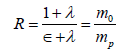(2)

Other mean parameter is the specific impulse, that describes the energy content of the propellant in a practical measure for use in engineering, in units of time (seconds), so this one provides the possibility of converting chemical energy into mechanical energy in terms of thrust, associate the correct nozzle design and engine, therefore, it is also the thrust developed per unit weight of propellant consumed in the time unit, as shown in Equation 3.

Δv.Ve (3)

Ve=Mo.M1 (4)

Where:

Δv: Maximum speed change

Ve: Total velocity

Mo: Total Mass

M1: Final Mass

Isp: Specific Impulse

The last equation show specify values you having in each stage, according to Tsiolkovsky rocket equation (4), which explains how the rocket acceleration subject to mass expulsion of propellant achieves high speeds from the conservation of momentum .

So, in a rocket due to atmospheric effects, the specific impulse varies with altitude, in the emptiness. That’s mean, the specific impulse is empty or under sea level .

This definition comes from knowing the burn rate depending on the specific impulse determined by the type of propellant used to further reduce the burning time is needed to achieve gravitational escape velocity, thus ensuring the minimum burn time.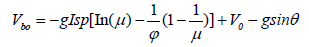(5)

Where the variables are meaning:

Vbo: Burnout Velocity.

mo: Initial mass in the phase

mf: Final mass in the phase

μ: Mass relation between initial and final mass.

θ: Flight angle

g0: Gravity in the sea level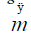: Propellant mass flow

me: Effective mass.

In addition, we need to consider the orbital velocity which is defined as the minimum speed that has a satellite, planet or body to orbit another body that is in the space, is set considering this factor is decisive when it comes to moving bodies, in that case it is to talk of a lower apogee height 350 km, in an elliptical path.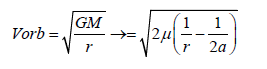(6)

Finally the most important factors to keep in consideration, they’re the flight angle and drag angle. In order to achieve greater control over the shape of the path, is necessary to make the Lift like negative, this means that the forces that act on the ascent will be the thrust and drag, and the lift will go towards the center of curvature path.

Clearly, the initial portion of the path must be nearly vertical, so that the length of the flight path on the rocket is subject to appreciable friction is reduced as much as possible. On the other hand, if it is too steep there will be an adverse effect on the speed due to the presence of gravity [7,8].

A variable angle of inclination is required “α”as a component of thrust along the path, in order to determine the best path.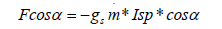(7)

Where we can find that:

F: Rocket ascendents force.

gs: Gravity in each phase in termins of height.

ISP: Specific impulse

m: Propelent’s mass flow.

Coasting describes the dynamic law, is meaning, the motion of a particle in a conservative field of force, and should always be such that the total energy and the angular momentum is conserved.

The changes that occur after Coasting are denoted as changes in speed, where the fixed coordinate system is denoted in polar coordinates.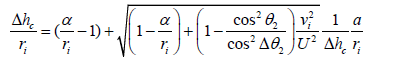(8)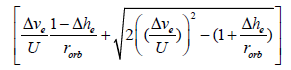This height defines us at what point you run out of rocket propellant, so this point is important to know the height limit of the stage.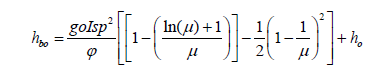(9)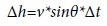(10)

h: Height Burnout

mb Gravity at sea level

tp: Specific Impulse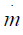: Relationship initial and final Mass

mo: Initial Height

Finally the whole trajectory is defined because they involve all the conditions to which it is subjected as the rocket thrust, speed, mass change, drag, earth rotation and change of gravity with altitude.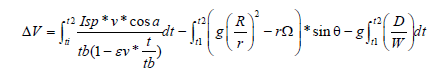(11)

The flight angle change over time relates the contribution of rocket thrust and the contribution of the Earth's rotation, changing speed and angle for the instantaneous values of these variables at each point of the trajectory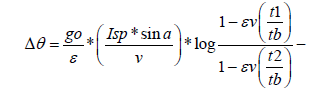(12)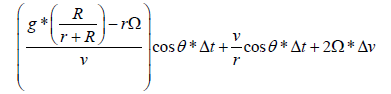When the rocket is launched since the Ecuador, the gross weight required is approximately 30 % less than if it had been presented at one of the poles. The launch in Ecuador also has an important advantage, because if you throw in the equatorial plane will be no north-south component of the Coriolis force.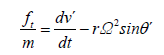(13)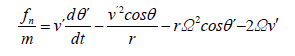(14)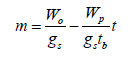(15)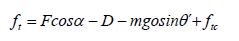(16)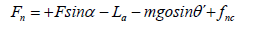(17)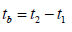(18)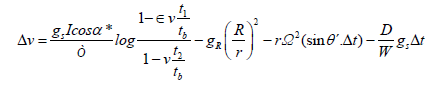(19)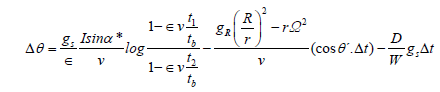(20)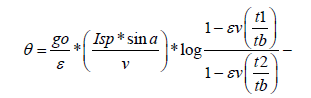(21)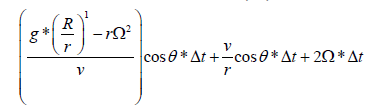With tilding factor that allows finding the reference values to know the behavior in the path unoptimized.

Where:

A: Angle with respect to the vertical

ε: Coefficient Structural

Dh: Changing height

ISP: Specific Impulse

v: Speed

θ: angle of flight

Ω: angular velocity of the Earth

Dt: Changing time

Also, it's necessary consider the right coordinates in the rotation system, in which the rocket is in favor of the rotation's direction of the earth, in which the relative speed increase and the rocket reached orbit speed every time in polar coordinate system type because they allow determining the spatial position of a specific point by a distance and two angles.

So the modeling patch is completed by integral equations of motion. With this, is possible verified that for each stage to follow, the curve defined ensures that an optimization is obtained. Through the optimization of integral equations of motion is performed by Euler's method; the solution is made to be increasing step of the independent variable in this case and thus a time derivative of this is assuming that no time variation is small and thus an approximation of the speed values is in terms of flight angle and height.

If the value of the variation is large they are can have large errors, but having a small variation values become very accurate.

#### Graphical Representation

The Figures 2-7 illustrates the main p arameters defined by the study of the orbit. Also take in account that

• The thrust is considering constant because a variation in the thrust force can be modified in order to ensure that during the stage there will be a delta (change), thrust altering the conditions of speed, altitude or flight angle the trajectory.

• The value of load factor is given by the pressure and mass for each of the stages. This value takes into account how it is affected by aerodynamic forces (Lift and drag).

• Payload ratio "λ" should be small in order to ensure optimal trajectory recalling that the objective is to calculate the masses (mass of propellant and structure).

Speed gas output results

Since speed is one of the main parameters to which the rocket is subject in each of the stages, the ideal speed of the gas outlet is calculated, the initial velocity is indicated as rocket behavior as it consumes propellant, this allows identifying the time available before the rocket thrust stop performing.

First stage: There is an increase in speed as it ascends the rocket.

Second stage: there is reached at increasing speed above the orbiting, then, the friction and the atmosphere cause a decrease in the speed.

Third stage: It is not reached deceleration counter friction propulsion then acceleration is less than the deceleration, as this speed is lower orbiting speed is reached.

Then, it is possible to determine how high the steps came and if the sum of which is greater than the height of perigee and after that, it is possible to determinate the states that the rocket would go into orbit. The sum of the three height increases show that the reported Cut off the end, considering that this must meet the orbital height. Also, Tilting is a value of an analysis does not take into account aerodynamic forces that is not a major change but if you want to know the behavior unoptimized path it is an interesting fact.

Optimal velocity

Speed starts decreasing at stage 3 and since it is subject to effects of air resistance, air friction, at the time of start of coasting the speed decreases, so the speed of the rocket is equal to the orbiting velocity. The rocket flight angle decreases as time passes. The change introduced to the first stage is achieved shortly before the rocket reaches the burn point but in steps two and three are present much earlier. Set if the height reached by the rocket as time passes gets the value of the optimal time for each stage thus fulfilling the stated values of the flight speed versus time.

#### Conclusion

The analysis of the trajectory of a rocket has different processes to ensure that the defined space mission is fulfilled, so it is necessary to conduct a thorough investigation directly to the rocket structurally affected, since the main parameter will be the structure of the rocket. This research does not account with profound gravity parameters and flight dynamics. Also coasting establish for each of the stages is necessary for predict the fall instead of each structure of the different stages, this being an important parameter when the launch site is selected.

They not currently have facilities in Colombia to support a space mission, but as seen in the development of the research, Colombia has a suitable space for the launch of a spacecraft due to its actual condition, about the latitudes and involvement rotation of land given by the Coriolis Effect. Through when the complete study of the temperature, pressure and density of the atmosphere at extreme altitudes is performed, the data can vary considerably. Especially the engine work.

#### References

Select your language of interest to view the total content in your interested language

### Article Usage

• Total views: 9771
• [From(publication date):
June-2016 - Jan 21, 2020]
• Breakdown by view type
• HTML page views : 9570Can't read the image? click here to refresh